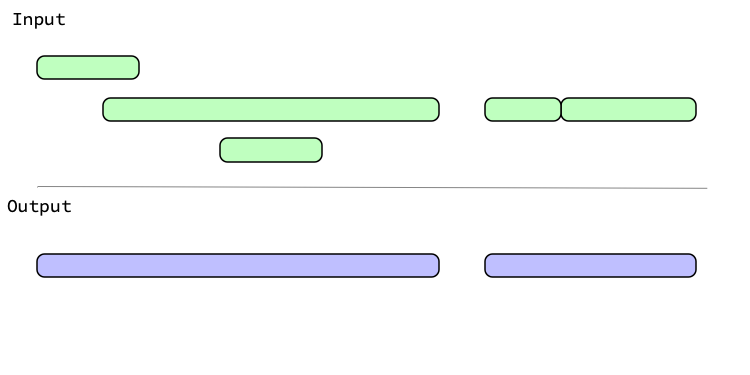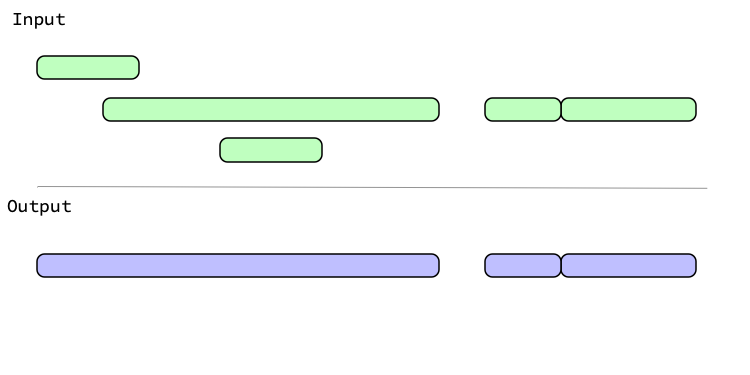This family of functions revolves around grouping overlapping intervals within a single iv. When multiple overlapping intervals are grouped together they result in a wider interval containing the smallest iv_start() and the largest iv_end() of the overlaps.

• iv_groups() merges all overlapping intervals found within x. The resulting intervals are known as the "groups" of x.

• iv_identify_group() identifies the group that the current interval of x falls in. This is particularly useful alongside dplyr::group_by().

• iv_locate_groups() returns a two column data frame with a key column containing the result of iv_groups() and a loc list-column containing integer vectors that map each interval in x to the group that it falls in.

Optionally, you can choose not to group abutting intervals together with abutting = FALSE, which can be useful if you'd like to retain those boundaries.

### Minimal interval vectors

iv_groups() is particularly useful because it can generate a minimal interval vector, which covers the range of an interval vector in the most compact form possible. In particular, a minimal interval vector:

• Has no overlapping intervals

• Has no abutting intervals

• Is ordered on both start and end

A minimal interval vector is allowed to have a single missing interval, which is located at the end of the vector.

## Usage

iv_groups(x, ..., abutting = TRUE)

iv_identify_group(x, ..., abutting = TRUE)

iv_locate_groups(x, ..., abutting = TRUE)

## Arguments

x

[iv]

An interval vector.

...

These dots are for future extensions and must be empty.

abutting

[TRUE / FALSE]

Should abutting intervals be grouped together?

If TRUE, [a, b) and [b, c) will merge as [a, c). If FALSE, they will be kept separate. To be a minimal interval vector, all abutting intervals must be grouped together.

## Value

• For iv_groups(), an iv with the same type as x.

• For iv_identify_group(), an iv with the same type and size as x.

• For iv_locate_groups(), a two column data frame with a key column containing the result of iv_groups() and a loc list-column containing integer vectors.

## Graphical Representation

Graphically, generating groups looks like:With abutting = FALSE, intervals that touch aren't grouped:## Examples

library(dplyr, warn.conflicts = FALSE)

x <- iv_pairs(
c(1, 5),
c(2, 3),
c(NA, NA),
c(5, 6),
c(NA, NA),
c(9, 12),
c(11, 14)
)
x
#> <iv<double>>
#>  [1, 5)   [2, 3)   [NA, NA) [5, 6)   [NA, NA) [9, 12)  [11, 14)

# Grouping removes all redundancy while still covering the full range
# of values that were originally represented. If any missing intervals
# are present, a single one is retained.
iv_groups(x)
#> <iv<double>>
#>  [1, 6)   [9, 14)  [NA, NA)

# Abutting intervals are typically grouped together, but you can choose not
# to group them if you want to retain those boundaries
iv_groups(x, abutting = FALSE)
#> <iv<double>>
#>  [1, 5)   [5, 6)   [9, 14)  [NA, NA)

# iv_identify_group() is useful alongside group_by() and summarize()
df <- tibble(x = x)
df <- mutate(df, u = iv_identify_group(x))
df
#> # A tibble: 7 × 2
#>           x         u
#>   <iv<dbl>> <iv<dbl>>
#> 1    [1, 5)    [1, 6)
#> 2    [2, 3)    [1, 6)
#> 3  [NA, NA)  [NA, NA)
#> 4    [5, 6)    [1, 6)
#> 5  [NA, NA)  [NA, NA)
#> 6   [9, 12)   [9, 14)
#> 7  [11, 14)   [9, 14)

df %>%
group_by(u) %>%
summarize(n = n())
#> # A tibble: 3 × 2
#>           u     n
#>   <iv<dbl>> <int>
#> 1    [1, 6)     3
#> 2   [9, 14)     2
#> 3  [NA, NA)     2

# The real workhorse here is iv_locate_groups(), which returns
# the groups and information on which observations in x fall in which
# group
iv_locate_groups(x)
#>        key     loc
#> 1   [1, 6) 1, 2, 4
#> 2  [9, 14)    6, 7
#> 3 [NA, NA)    3, 5